## 6.2 Lewis Structures

### Learning Objectives

By the end of this section, you will be able to:

• Write Lewis symbols for neutral atoms and ions
• Draw Lewis structures depicting the bonding in simple molecules

We have discussed the various types of bonds that form between atoms and/or ions. In all cases, these bonds involve the sharing or transfer of valence shell electrons between atoms. In this section, we will explore the typical method for depicting valence shell electrons and chemical bonds, namely Lewis symbols and Lewis structures.

## Lewis Symbols

We use Lewis symbols to describe valence electron configurations of atoms and monatomic ions. A Lewis symbol consists of an elemental symbol surrounded by one dot for each of its valence electrons: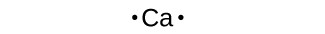Figure 1 shows the Lewis symbols for the elements of the third period of the periodic table.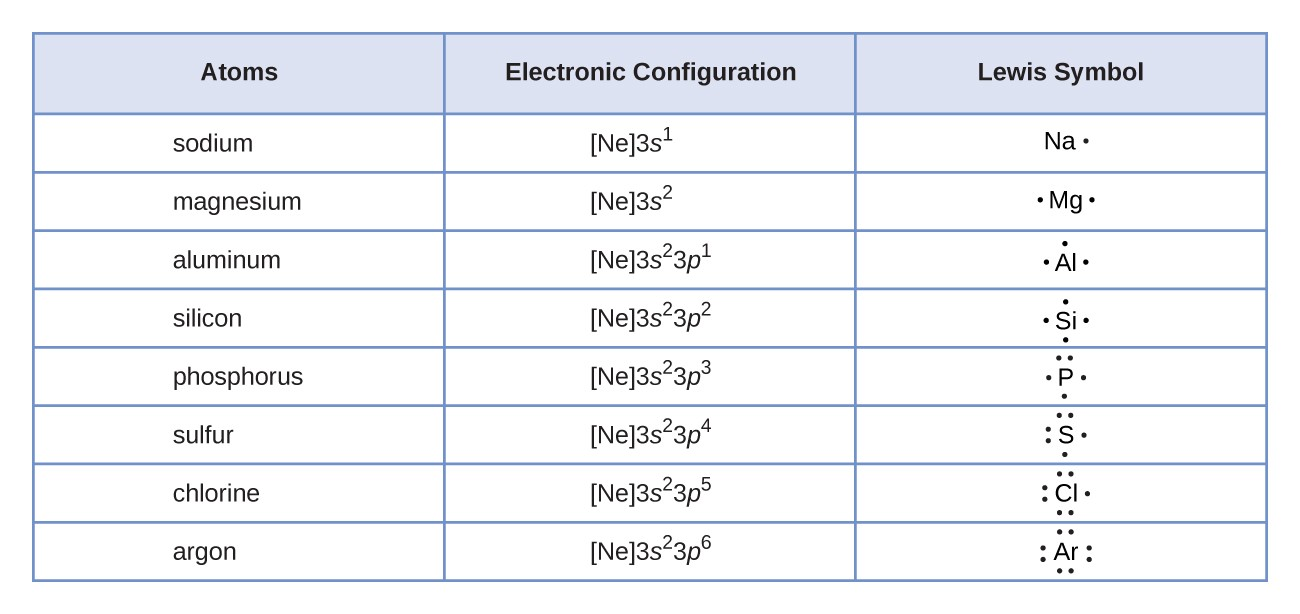Figure 1. Lewis symbols illustrating the number of valence electrons for each element in the third period of the periodic table.

Lewis symbols can also be used to illustrate the formation of cations from atoms, as shown here for sodium and calcium:Likewise, they can be used to show the formation of anions from atoms, as shown below for chlorine and sulfur: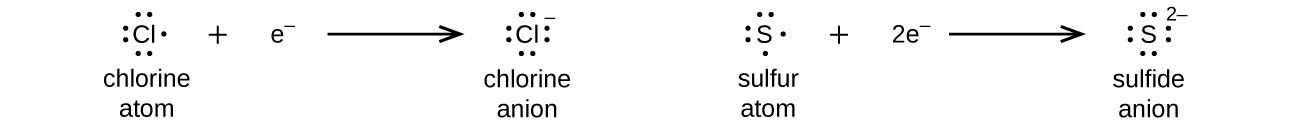Figure 2 demonstrates the use of Lewis symbols to show the transfer of electrons during the formation of ionic compounds.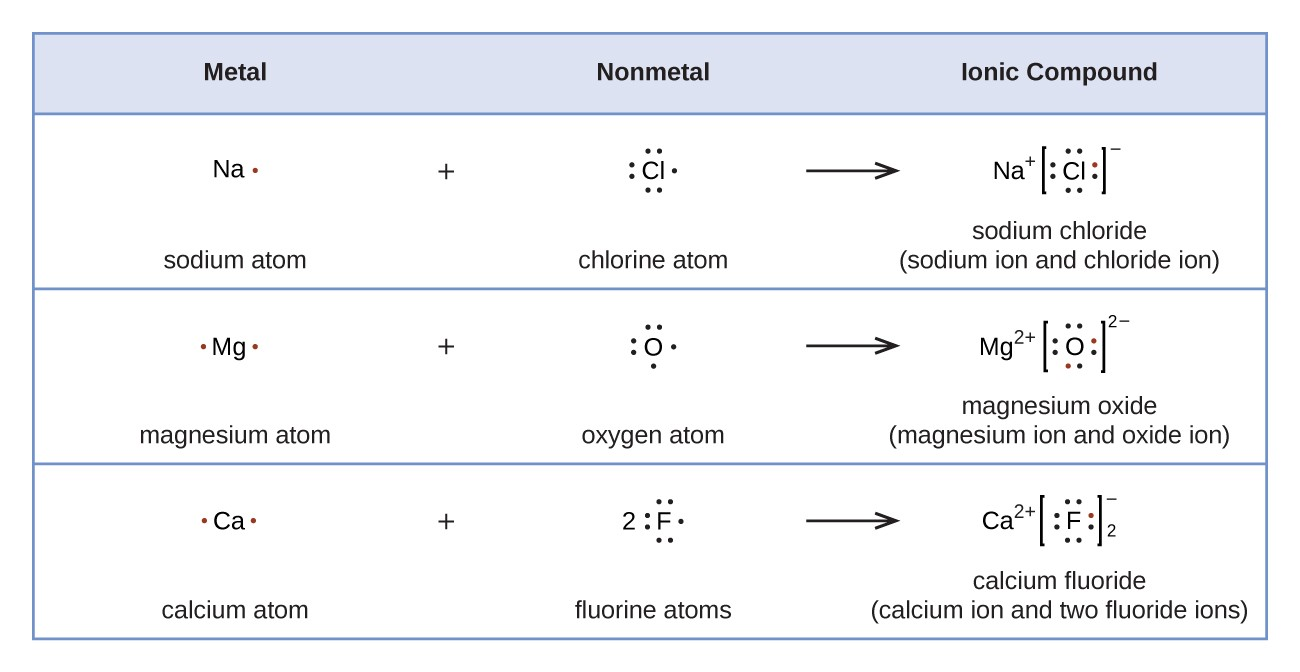Figure 2. Cations are formed when atoms lose electrons, represented by fewer Lewis dots, whereas anions are formed by atoms gaining electrons. The total number of electrons does not change.

## Lewis Structures

We also use Lewis symbols to indicate the formation of covalent bonds, which are shown in Lewis structures, drawings that describe the bonding in molecules and polyatomic ions. For example, when two chlorine atoms form a chlorine molecule, they share one pair of electrons: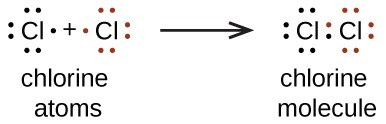The Lewis structure indicates that each Cl atom has three pairs of electrons that are not used in bonding (called lone pairs) and one shared pair of electrons (written between the atoms). A dash (or line) is sometimes used to indicate a shared pair of electrons: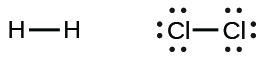A single shared pair of electrons is called a single bond. Each Cl atom interacts with eight valence electrons: the six in the lone pairs and the two in the single bond.

### The Octet Rule

The other halogen molecules (F2, Br2, I2, and At2) form bonds like those in the chlorine molecule: one single bond between atoms and three lone pairs of electrons per atom. This allows each halogen atom to have a noble gas electron configuration. The tendency of main group atoms to form enough bonds to obtain eight valence electrons is known as the octet rule.

The number of bonds that an atom can form can often be predicted from the number of electrons needed to reach an octet (eight valence electrons); this is especially true of the nonmetals of the second period of the periodic table (C, N, O, and F). For example, each atom of a group 14 element has four electrons in its outermost shell and therefore requires four more electrons to reach an octet. These four electrons can be gained by forming four covalent bonds, as illustrated here for carbon in CCl4 (carbon tetrachloride) and silicon in SiH4 (silane). Because hydrogen only needs two electrons to fill its valence shell, it is an exception to the octet rule. The transition elements and inner transition elements also do not follow the octet rule: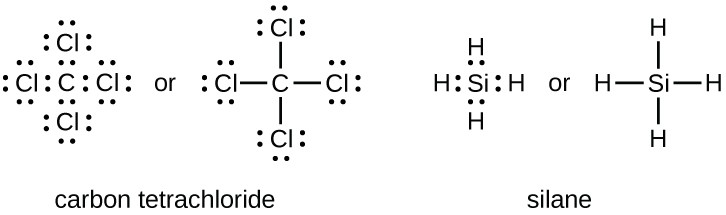Group 15 elements such as nitrogen have five valence electrons in the atomic Lewis symbol: one lone pair and three unpaired electrons. To obtain an octet, these atoms form three covalent bonds, as in NH3 (ammonia). Oxygen and other atoms in group 16 obtain an octet by forming two covalent bonds: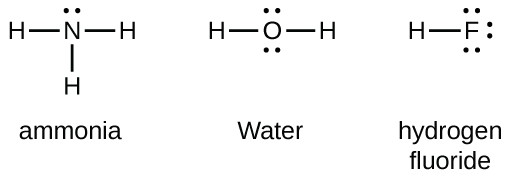### Double and Triple Bonds

As previously mentioned, when a pair of atoms shares one pair of electrons, we call this a single bond. However, a pair of atoms may need to share more than one pair of electrons in order to achieve the requisite octet. A double bond forms when two pairs of electrons are shared between a pair of atoms, as between the carbon and oxygen atoms in CH2O (formaldehyde) and between the two carbon atoms in C2H4 (ethylene):A triple bond forms when three electron pairs are shared by a pair of atoms, as in nitrogen gas (N2):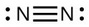## Writing Lewis Structures with the Octet Rule

For very simple molecules and molecular ions, we can write the Lewis structures by merely pairing up the unpaired electrons on the constituent atoms. See these examples: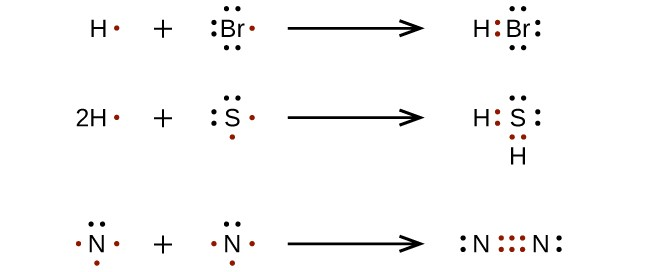For more complicated molecules and molecular ions, it is helpful to follow the step-by-step procedure outlined here:

1. Determine the total number of valence (outer shell) electrons.
2. Draw a skeleton structure of the molecule, arranging the atoms around a central atom. (Generally, the atom that can form more bonding pairs will be the central element, or as we will learn later, the least electronegative element will be the central atom.) Connect each atom to the central atom with a single bond (one electron pair).
3. Distribute the remaining electrons as lone pairs on the terminal atoms (except hydrogen), completing an octet around each atom.
4. Place all remaining electrons on the central atom.
5. Rearrange the electrons of the outer atoms to make multiple bonds with the central atom in order to obtain octets wherever possible.

Let us determine the Lewis structure of PBr3 using the steps above:

• Step 1: Determine the total number of valence (outer shell) electrons.

$\large \begin{array}{l}\\ \phantom{\rule{0.8em}{0ex}}{\text{PBr}}_{3}\\ \phantom{\rule{0.8em}{0ex}}\text{P: 5 valence electrons/atom}\times \text{1 atom}=5\\ \underline{+\text{Br: 7 valence electron/atom}\times \text{3 atoms}=21}\\ \\ \phantom{\rule{15.95em}{0ex}}=\text{26 valence electrons}\end{array}$

• Step 2: Draw a skeleton structure of the molecule, arranging the atoms around a central atom. (Generally, the least electronegative element should be placed in the center.) Connect each atom to the central atom with a single bond (one electron pair).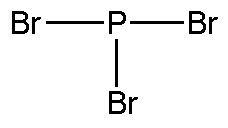• Step 3: Distribute the remaining electrons as lone pairs on the terminal atoms (except hydrogen), completing an octet around each atom.
•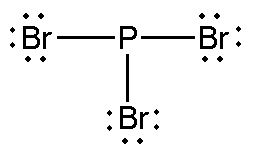Step 4: Place all remaining electrons on the central atom.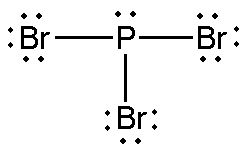Note: Step 5: Is not needed since all atoms have an octet.

Let us determine the Lewis structure of CH2O.

• Step 1: Determine the total number of valence (outer shell) electrons.

$\large \begin{array}{l}\\ \phantom{\rule{0.8em}{0ex}}{\text{H}_{2}}\text{CO}\\ \phantom{\rule{0.8em}{0ex}}\text{H: 1 valence electron/atom}\times \text{2 atom}=2\\\text{C: 4 valence electrons/atom}\times \text{1 atom}=4\\ \underline{+\text{O: 6 valence electrons/atom}\times \text{1 atoms}=6}\\ \\ \phantom{\rule{15.95em}{0ex}}=\text{12 valence electrons}\end{array}$

• Step 2: Draw a skeleton structure of the molecule, arranging the atoms around a central atom. (Generally, the least electronegative element should be placed in the center.) Connect each atom to the central atom with a single bond (one electron pair).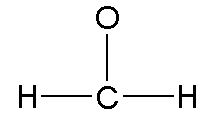• Step 3: Distribute the remaining electrons as lone pairs on the terminal atoms (except hydrogen), completing an octet around each atom.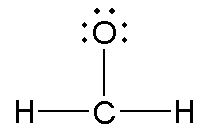• Step 4: Not needed, since all electrons have been placed.  However, carbon does not have an octet,
• Step 5: Rearrange the electrons of the outer atoms to make multiple bonds with the central atom in order to obtain octets wherever possible.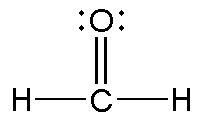### Example 1: Writing Lewis Structures

NASA’s Cassini-Huygens mission detected a large cloud of toxic hydrogen cyanide (HCN) on Titan, one of Saturn’s moons. What are the Lewis structures of these molecules?

Carbon dioxide, CO2, is a product of the combustion of fossil fuels. CO2 has been implicated in global climate change. What is the Lewis structure of CO2?

### Key Concepts and Summary

Valence electronic structures can be visualized by drawing Lewis symbols (for atoms and monatomic ions) and Lewis structures (for molecules and polyatomic ions). Lone pairs, unpaired electrons, and single, double, or triple bonds are used to indicate where the valence electrons are located around each atom in a Lewis structure. Most structures—especially those containing second row elements—obey the octet rule, in which every atom (except H) is surrounded by eight electrons. Exceptions to the octet rule occur for odd-electron molecules (free radicals), electron-deficient molecules, and hypervalent molecules.

### Exercises

1. Write the Lewis symbols for each of the following ions:
1. As3–
2. I
3. Be2+
4. O2–
5. Ga3+
6. Li+
7. N3–
2. Many monatomic ions are found in seawater, including the ions formed from the following list of elements. Write the Lewis symbols for the monatomic ions formed from the following elements:
1. Cl
2. Na
3. Mg
4. Ca
5. K
6. Br
7. Sr
8. F
3. Write the Lewis symbols of the ions in each of the following ionic compounds and the Lewis symbols of the atom from which they are formed:
1. MgS
2. Al2O3
3. GaCl3
4. K2O
5. Li3N
6. KF
4. In the Lewis structures listed below, M and X represent various elements in the third period of the periodic table. Write the formula of each compound using the chemical symbols of each element:
1.2.3.4.5. Write the Lewis structure for the diatomic molecule P2, an unstable form of phosphorus found in high-temperature phosphorus vapor.
6. Write Lewis structures for the following:
1. H2
2. HBr
3. PCl3
7. Write Lewis structures for the following:
1. O2
2. H2CO
3. AsF3
4. SiCl4

## Glossary

double bond: covalent bond in which two pairs of electrons are shared between two atoms

free radical: molecule that contains an odd number of electrons

hypervalent molecule: molecule containing at least one main group element that has more than eight electrons in its valence shell

Lewis structure: diagram showing lone pairs and bonding pairs of electrons in a molecule or an ion

Lewis symbol: symbol for an element or monatomic ion that uses a dot to represent each valence electron in the element or ion

lone pair: two (a pair of) valence electrons that are not used to form a covalent bond

octet rule: guideline that states main group atoms will form structures in which eight valence electrons interact with each nucleus, counting bonding electrons as interacting with both atoms connected by the bond

single bond: bond in which a single pair of electrons is shared between two atoms

triple bond: bond in which three pairs of electrons are shared between two atoms